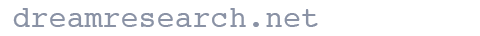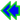(See the bottom of the page for an example of the use of this table.)

#### Critical h values for confidence levels of 5% and 1%

N or N'critical h
p=.05p=.01
15.716.941
16.693.911
17.672.884
18.653.859
19.636.836
20.620.815
21.605.795
22.591.777
23.578.760
24.566.744
25.554.729
26.544.714
27.533.701
28.524.688
29.515.676
30.506.665
31.498.654
32.490.644
33.483.634
34.475.625
35.469.616
N or N'critical h
p=.05p=.01
36.462.607
37.456.599
38.450.591
39.444.583
40.438.576
42.428.562
44.418.549
46.409.537
48.400.526
50.392.515
52.384.505
54.377.496
56.370.487
58.364.478
60.358.470
64.346.455
68.336.442
72.327.429
76.318.418
80.310.407
84.302.397
N or N'critical h
p=.05p=.01
88.295.388
92.289.380
96.283.372
100.277.364
120.253.333
140.234.308
160.219.288
180.207.272
200.196.258
250.175.230
300.160.210
350.148.195
400.139.182
450.131.172
500.124.163
550.118.155
600.113.149
700.105.138
800.098.129
900.092.121
1000.088.115
Formulas used to find these h values:
For p=.05:For p=.01:To illustrate the use of this table, let us assume that we are comparing two samples each having 140 observations in them and an h difference of .32. Since the N in both samples is the same, we look down the column marked N or N' until we come to 140. We look to our right and see that an h of .23 or above is significant at the .05 level of confidence and an h of .31 is significant at the .01 level. Since the h in this hypothetical example is .32, the difference between the two samples is statistically significant at the .01 level of confidence, meaning there is less than one chance in a hundred that the difference we have found is not a real difference.

Since it is widely known that a Z-score of 1.96 is significant at the .05 level of confidence and one of 2.58 significant at the .01 level, some researchers might want to display the significance finding in terms of a Z score. There is an easy formula for finding Z from h: Z is h times the square root of n/2.

In the above example, the N for both samples was the same. In many cases, however, the samples will be of different sizes. Then N' must be determined with the following straightforward formula, where n1 is one sample and n2 is the other: N' = (2*n1*n2)/(n1+n2)

Once N' is determined, it also can be used in the formula to convert h to Z: Z is h times the square root of N'/2.

As can be seen by a casual inspection of the above table, it does not take a very large h for statistical significance when sample sizes are in the hundreds. Since, as noted, our sample sizes are usually large, the question of statistical significance is not a primary one for us. Perhaps this table makes it even more clear why we are concerned with effect sizes rather than statistical significance.Go back to the Statistics page.